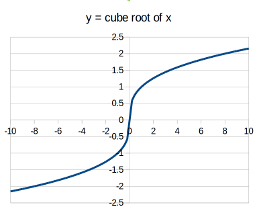The cube root of a number $x$ is a number $y$ such that $y^3=x.$

We write that $y$ is the cube root of $x$ thus: $y=\sqrt{x}.$

The cube root operation from real numbers to real numbers is a function and is one-to-one. Its derivative approaches $\infty$ as $x$ approaches $0,$ so the line is in some sense "vertical" at the point $(0,0)$ - the tangent line at $(0,0)$ is vertical.

BasinOfAttraction
FundamentalTheoremOfAlgebra
NewtonsMethod
RootsOfPolynomials
SquareRoot
(none) DomainOfAFunction
MathematicsTaxonomy
RationalisingTheDenominator
RealNumber
TypesOfNumber
Root AlgebraicNumber
Surd

## You are here

CubeRoot
Function
RealNumber
FundamentalTheoremOfAlgebra
NewtonsMethod
RiemannHypothesis
SquareRoot
(none) AlgebraicNumber
CauchySequence
CoDomainOfAFunction
ContinuedFraction
DedekindCut
DomainOfAFunction
ImageOfAFunction
IrrationalNumber
Polynomial
RangeOfAFunction
RationalNumber
TranscendentalNumber